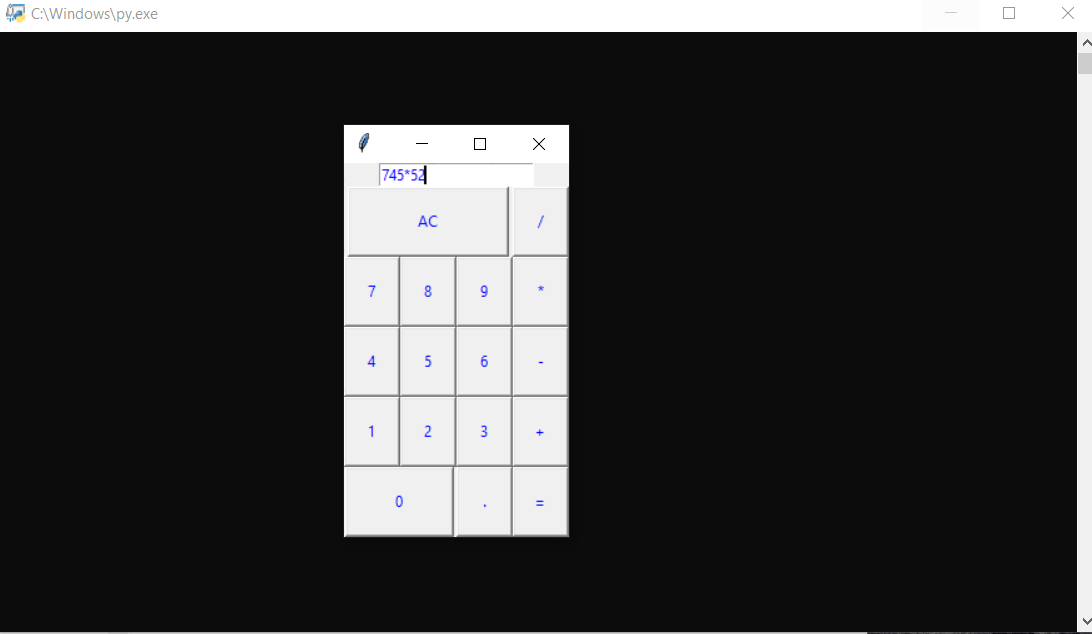## SIMPLE CALCULATOR IN PYTHON WITH SOURCE CODE

The Simple Calculator In Python is a simple project developed using Python. The project contains the numbers, operators, and sign like in normal calculator. The user can enter any number or can just click on the numbers they want in the calculations. Henc...

Type : Project

File Size : 1.2 KB# Similar Projects and Reports

#### SIMPLE CALCULATOR IN PYTHON WITH SOURCE CODE

The Simple Calculator In Python is a simple project developed using Python. The project contains the numbers, operators, and sign like in normal calculator. The user can enter any number or can just click on the numbers they want in the calculations. Henc...

#### Simple Calculator In GUI Python With Source Code

This Simple Calculator In GUI is a simple project developed using Python. The project contains the numbers, operators, and signs like on a normal calculator. Here, the user can just click on the numbers they want in the calculations. Hence, the user can u...

#### Simple Age Calculator In Python With Source Code

The Simple Age Calculator In Python is a simple project developed using Python. The project is for the user's convenience, to help them find out about their exact age in year, month, and days. The project file contains a python script ( AGE.py). This is a...

#### Simple GUI Calculator In Python With Source Code

The Simple GUI Calculator In Python is a simple project developed using Python. The project contains the numbers, operators, and signs like in a normal calculator. So, the user cannot enter the number themselves, they can just click on the numbers they wa...

#### Simple BMI Calculator In Python With Source Code

The Simple BMI Calculator In Python is a simple project developed using Python. The project is for the user convenience, for checking your BMI. With the BMI value, you can check whether you have a healthy weight or not. The project file contains a python...

#### CALCULATOR IN PYTHON WITH SOURCE CODE

This Calculator project In Python is a simple project developed using Python. The project contains the numbers, operators, and sign like in normal calculator. So, the user can enter any number they want in the calculations. Hence, the user can use a simpl...